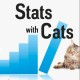## WHAT ARE THE ODDS?

Probability is the core of statistics. You hear the phrase “what’s the probability of …” all the time in statistics. You also hear that phrase in everyday life, too. What’s the probability of rain tomorrow? What’s the probability of winning the lottery? You also hear the phrase “what are the odds …? What are the odds my team will win the game? What are the odds I’ll catch the flu? It sounds just like probability, but it’s not.

Probability is defined as the number of favorable events divided by the total number of events. Probabilities range from 0 (0%) to 1 (100%). To convert from a probability to odds, divide the probability by one minus that probability.

Odds are defined as the number of favorable events divided by the number of unfavorable events. This is the same as saying that odds are defined as the probability that the event will occur divided by the probability that the event will not occur. Odds range from 0 to infinity. To convert from odds to a probability, divide the odds by one plus the odds.

A probability of 0 is the same as odds of 0. Probabilities between 0 and 0.5 equal odds less than 1.0. A probability of 0.5 is the same as odds of 1.0. As the probability goes up from 0.5 to 1.0, the odds increase from 1.0 to infinity.

Probability and odds are expressed in several ways, as a fraction, as a decimal, as a percentage, or in words. Words used to express probability are “out of” and “in.” For example, a probability could be expressed as 1/5, 0.2, 20%, 1 out of 5, or 1 in 5. The words used to express odds are “-” and “to.” For example, odds could be expressed as 1/4, 0.25, 25%, 1-4, or 1 to 4. There are even more ways to express odds for gambling, but you won’t have to know about them to pass Stats 101. In fact, you might not even hear about expressing uncertainty using odds in an introductory statistics course. Odds become important in more advanced statistics classes in which odds ratio and relative risk are discussed.

Odds, like probabilities, come from four sources—logic, data, oracles, and models. Perhaps the best-known examples of odds creation come from the oracles in Las Vegas. Those oracles, called bookmakers, decide what the odds should be for a particular bet. Historically, before computers, bookmakers would compile as much information as they could about prior events and conditions related to the bet, then make educated guesses about what might happen based on their experience, intuition, and judgment. With computers, this job has become bigger, faster, more complicated, and more reliant on statistics and technology. The field of sports statistics has exploded since the 1970s when baseball statistics (sabermetrics) emerged. Modern gambling odds even fluctuate to take account of wagers placed before the actual event. To keep up with the demand, bookmakers now employ teams of Traders who compile the data and use statistical models to estimate the odds. But you’ll never have to know that for Stats 101.

Next, the answer to the question “which came first, the model or the odds?”